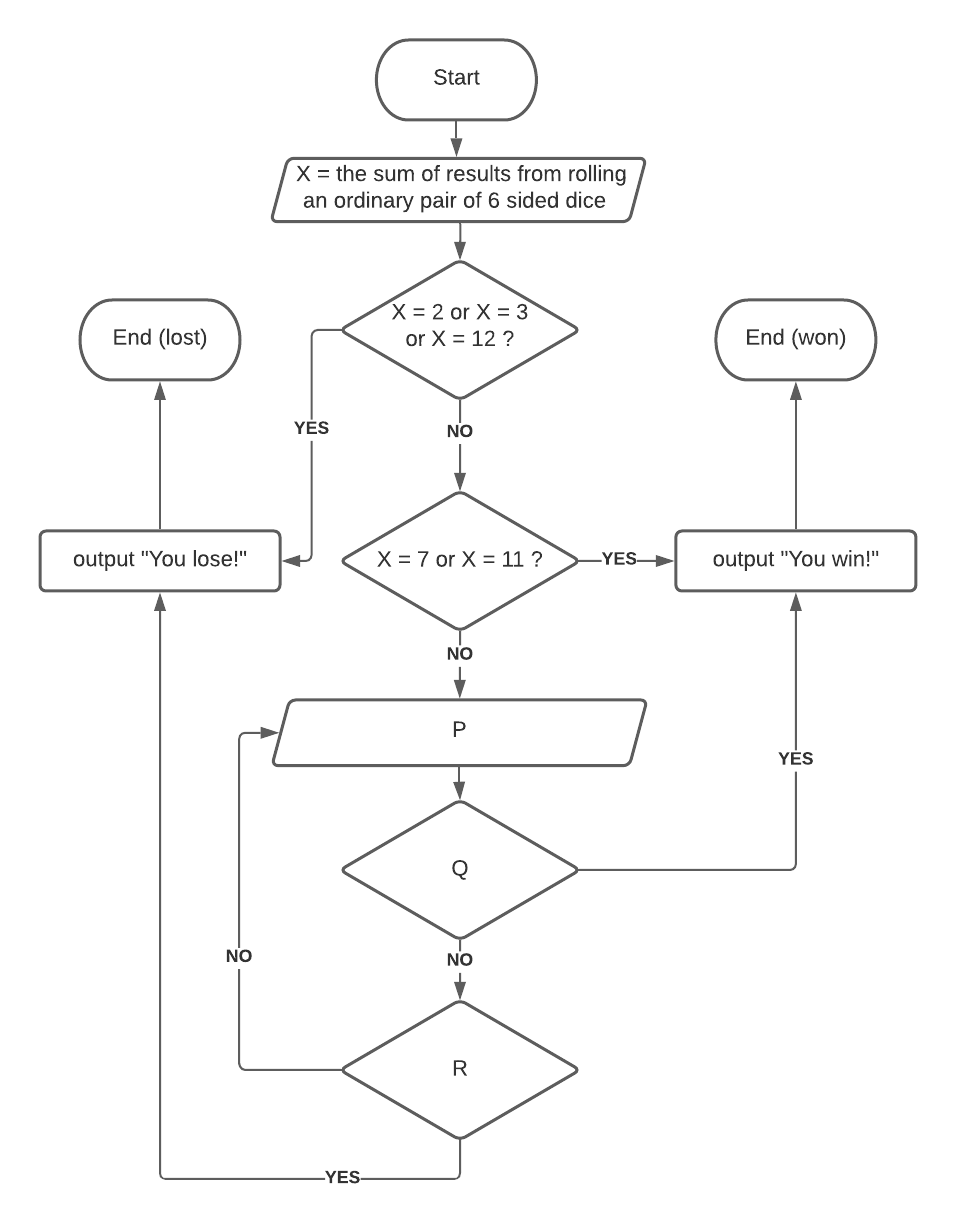Login or Create an Account to view the mark scheme, comment, and add to a test
Consider a game where two dice are rolled and the sum calculated. If a player rolls a sum of 2, 3, or 12 on the first roll, that player loses. If a player rolls a sum of 7 or 11 on the first roll, that player wins. Any other first roll sum becomes the player's new target to win. The player must keep rolling until they either roll their new target to win or a 7 to lose. For example, if a player rolls a 10 on the first roll, their target sum to win becomes 10 and they must keep rolling until they either roll a 10 to win or a 7 to lose. Select options for the items P, Q & R as indicated in the following flow diagram in order to represent the game logic as described.(a).
P: Y = the sum of results from rolling an ordinary pair of 6 sided dice Q: X = Y ? R: Y = 7 ?
(b).
P: X = Y ? Q: Y = the sum of results from rolling an ordinary pair of 6 sided dice R: Y = 7 ?
(c).
P: Y = the sum of results from rolling an ordinary pair of 6 sided dice Q: Y = 7 ? R: X = Y ?
(d).
P: Y = the sum of results from rolling an ordinary pair of 6 sided dice Q: Y = 10 ? R: Y = 7 ?

HomeThe Right Test - Fast